#Function Repository Resource:

# LongFormDataset

Converts tabular data into a long-form dataset

Contributed by: Anton Antonov
 ResourceFunction["LongFormDataset"][data] converts the argument data into a long-form dataset with columns "AutomaticKey","Variable" and "Value". ResourceFunction["LongFormDataset"][data, idcols] converts the argument data into a long-form dataset with columns idcols and columns "Variable" and "Value" derived from the columns of data that are not in idcols. ResourceFunction["LongFormDataset"][data, idcols, valcols] converts the argument data into a long-form dataset with columns idcols and columns "Variable" and "Value" derived from valcols.

## Details and Options

The so called "long form" and "wide form" conversions of tabular data are fundamental "data wrangling" operations.
In long form conversion, the columns of the original dataset are seen in three groups: identifier columns, variable columns and ignored columns.
The conversion into long form converts the set of specified variable columns into two columns: one with variable names and the other with variable values.
The first argument of ResourceFunction["LongFormDataset"] can be a two-dimensional (non-hierarchical) dataset, a two dimensional full array (matrix), a list of associations or an association of associations.
The conversion of a dataset into a long form allows column names (of variables) to be treated as data.
If no identifier columns are given, ResourceFunction["LongFormDataset"] uses the order indexes of the rows as identifiers.
ResourceFunction["LongFormDataset"] takes the following options:
 "AutomaticKeysTo" "AutomaticKey" the column name if automatic keys are used "VariablesTo" "Variable" the column name of the column that has the variable names as values "ValuesTo" "Value" the column name of the column that has the variable values as values

## Examples

### Basic Examples (4)

Here is a simple dataset:

 In:=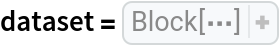Out=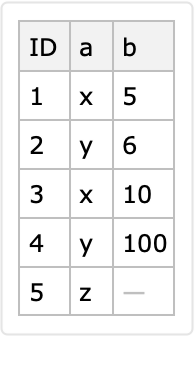Here is a long form conversion with a specified identifier column and variable columns:

 In:=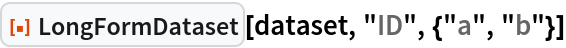Out=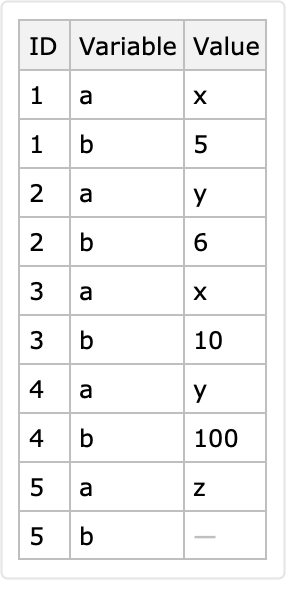Here is another long form derived with an automatically determined identifier column and variable columns:

 In:=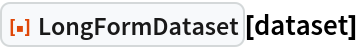Out=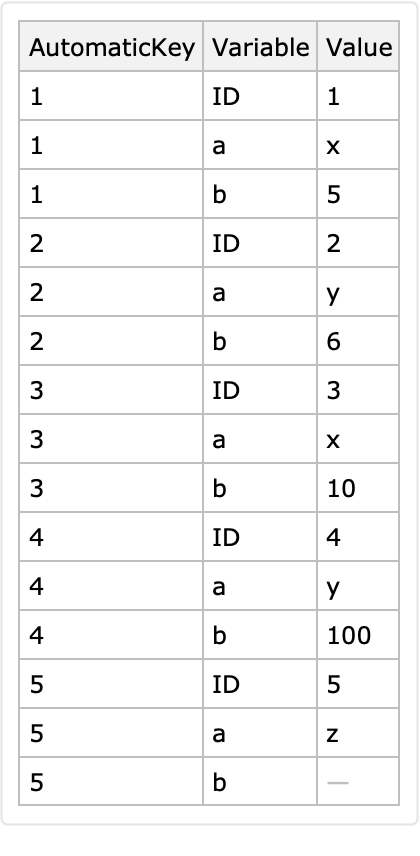Here is a conversion to long form with identifier columns "a" and "b":

 In:=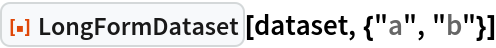Out=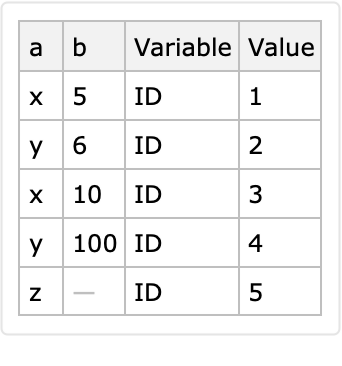### Scope (5)

The first argument can be a matrix:

 In:=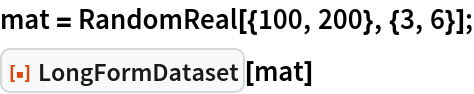Out=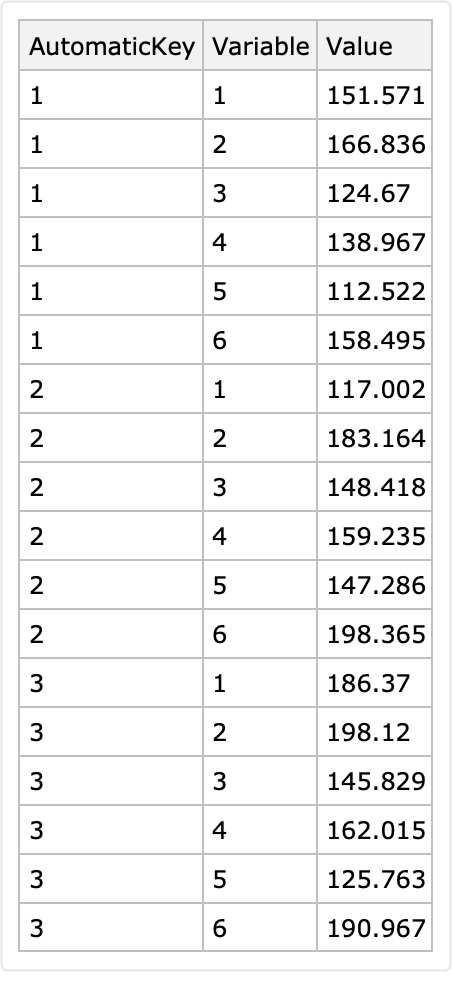The first argument can be a list of associations:

 In:=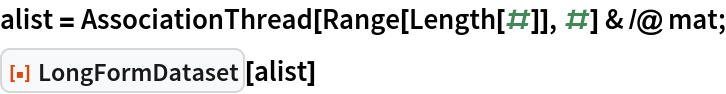Out=The second and third arguments can be Automatic:

 In:=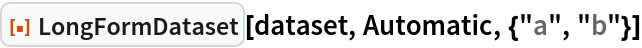Out=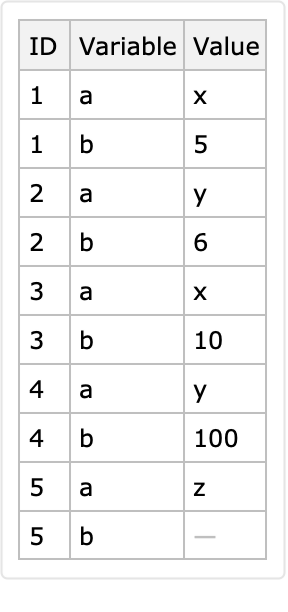In:=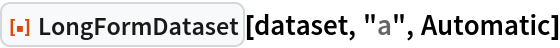Out=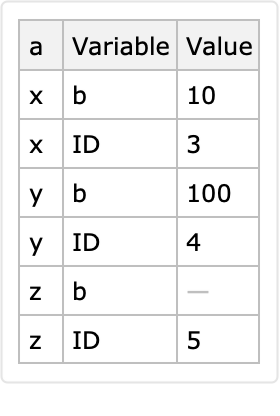The options "IdentifierColumns" and "VariableColumns" can be used instead of a second argument and third argument respectively:

 In:=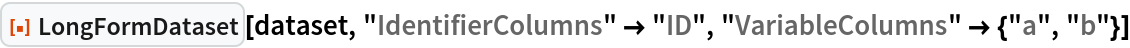Out=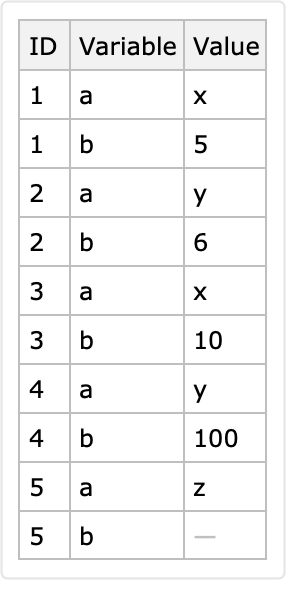The second and third arguments can be column indexes:

 In:=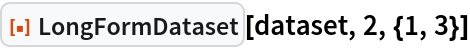Out=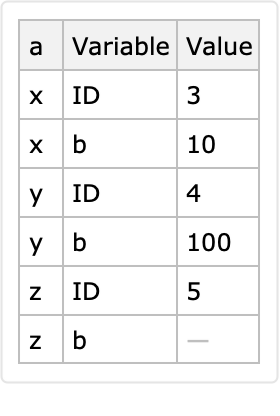Automatic keys are derived if the dataset argument has row keys:

 In:=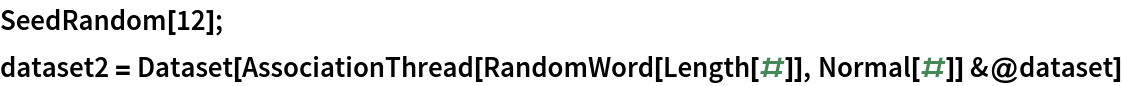Out=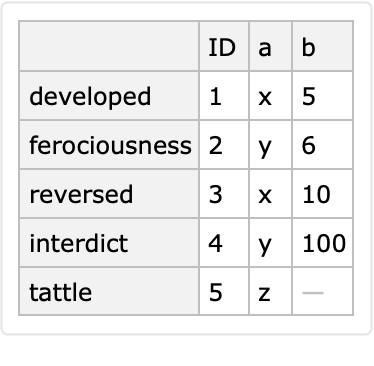Here is an automatic conversion to long form:

 In:=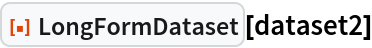Out=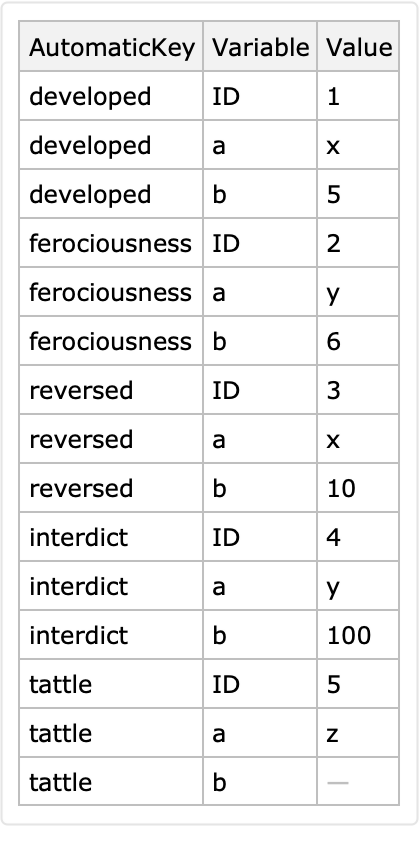The row keys are ignored if the identifier column is specified:

 In:=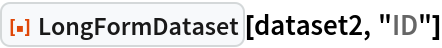Out=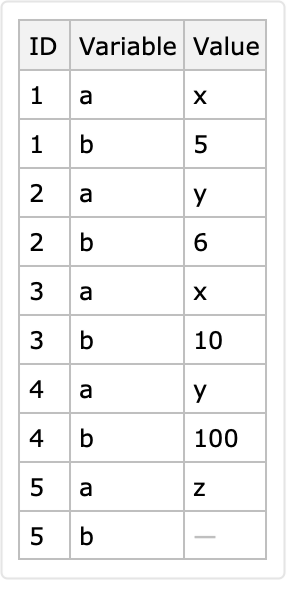### Options (3)

#### AutomaticKeysTo (1)

The option "AutomaticKeysTo" can be used to specify the name of the column that corresponds to the automatically determined identifier:

 In:=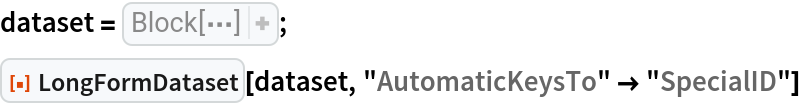Out=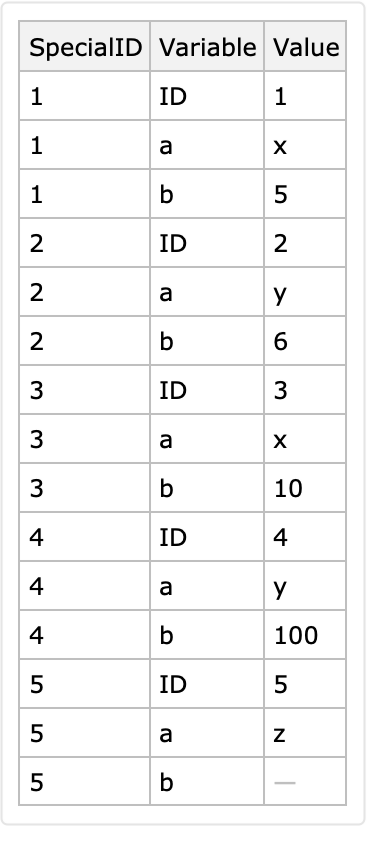#### VariablesTo (1)

The option "VariablesTo" specifies the name of long form's column that has, as values, the names of the variable columns:

 In:=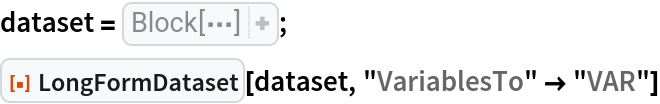Out=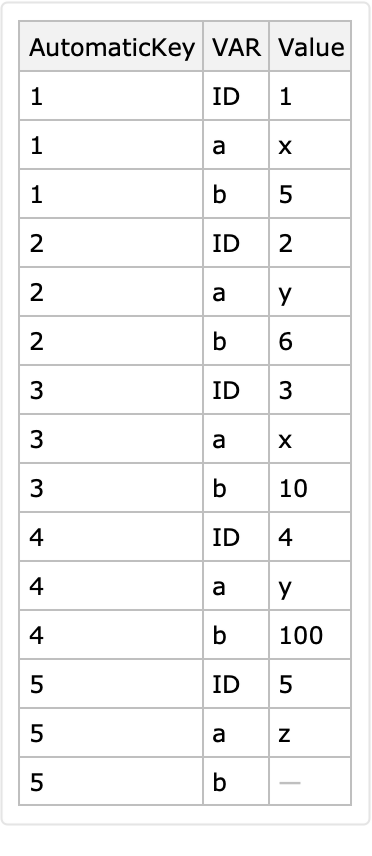#### ValuesTo (1)

The option "ValuesTo" specifies the name of the column that has the values in the variable columns:

 In:=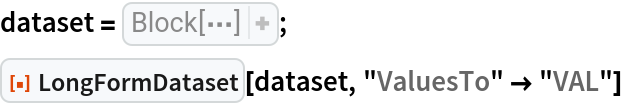Out=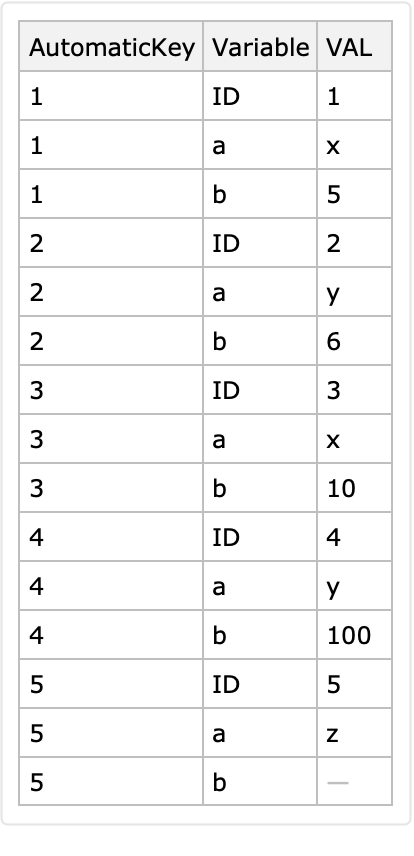### Applications (9)

#### Column names as data (5)

The main advantage of the long form conversion is that variable column names become data, that is, values in a certain column. This can be demonstrated by making an association of time series objects for the rows of multiple time series data given in wide form.

Here is a randomly generated table (dataset) with multiple time series data:

 In:=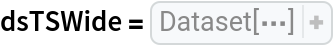Out=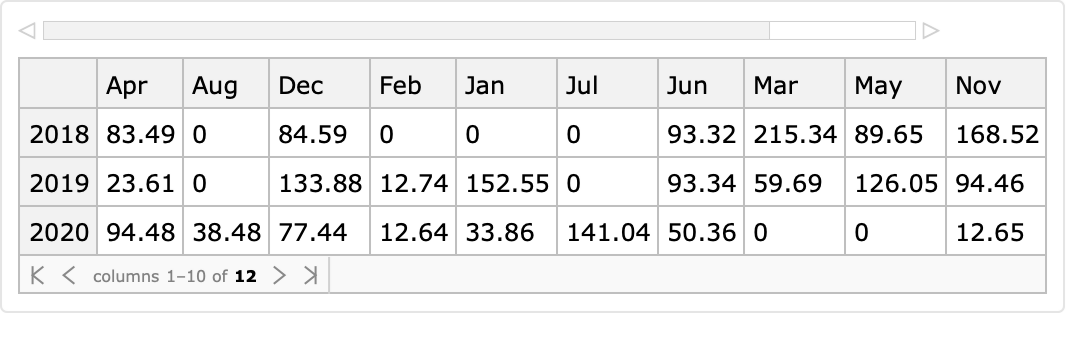Each row of that random time series dataset corresponds to a yearly sequence of monthly values. The interpretation of the dataset reveals heterogeneous semantics of its values: (1) each row corresponds to a year specified as a key and (2) the columns have short month names.

Convert the dataset into long form using the row keys as identifiers:

 In:=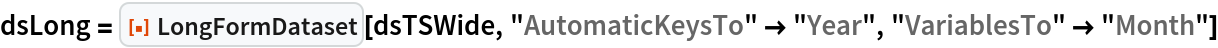Out=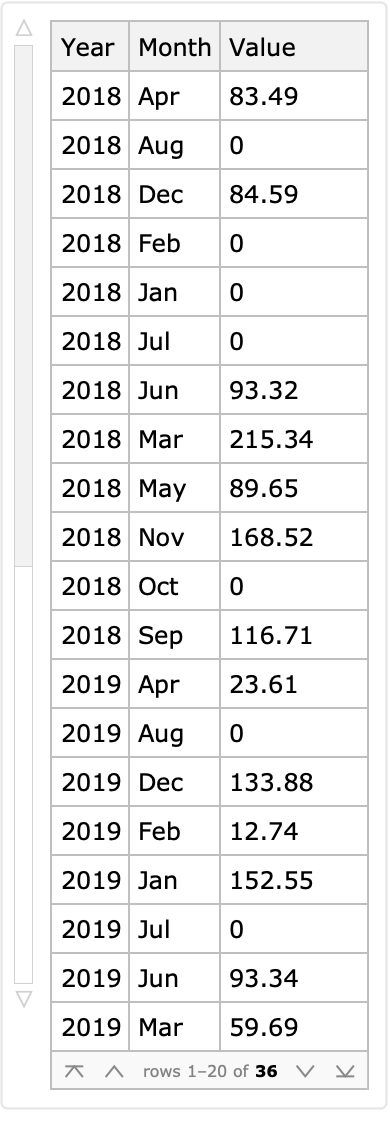Add the column "ObservationTime" derived from the columns "Year" and "Month":

 In:=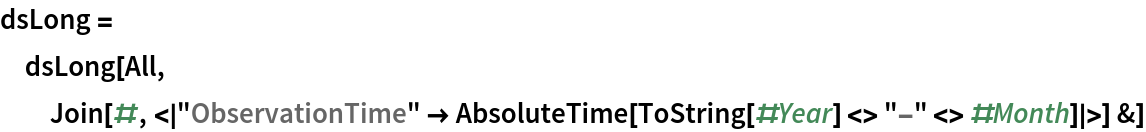Out=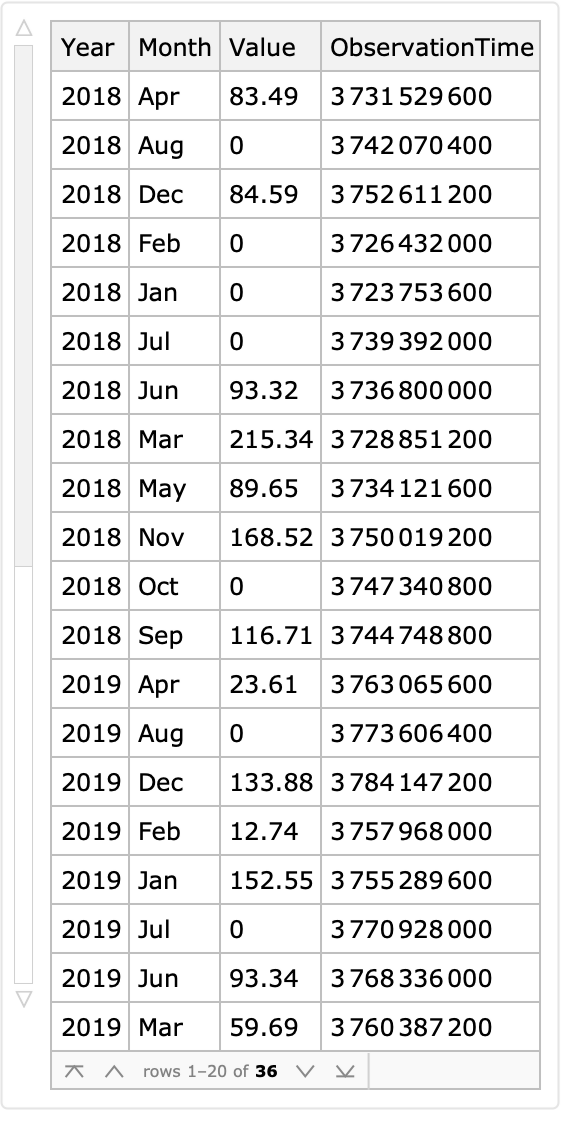Split the long form dataset by year and make time series with the columns "ObservationTime" and "Value":

 In:=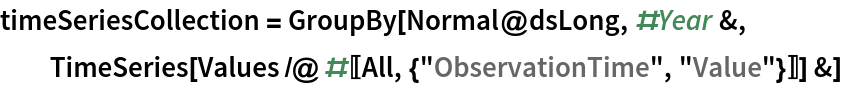Out=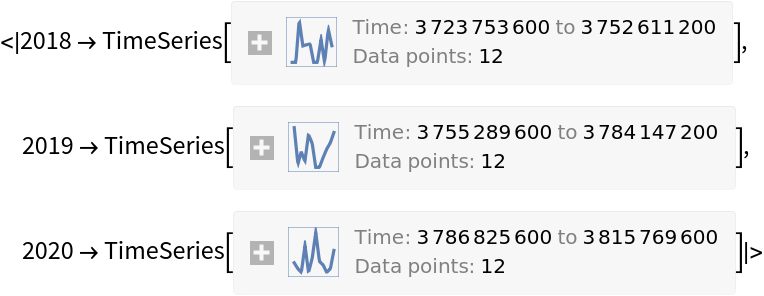Plot the obtained time series:

 In:=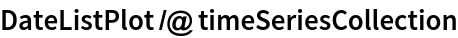Out=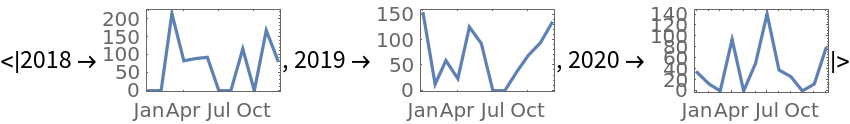#### Combinations of the heterogenous data (4)

Using long forms simplifies the programmatic manipulation of heterogenous data.

Get datasets with different numbers of rows and columns that correspond to items and variables of different kinds:

 In:=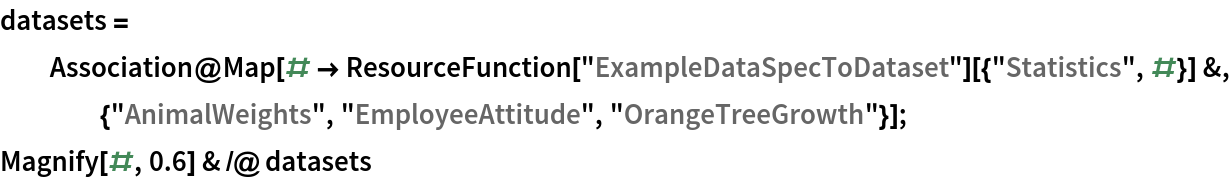Out=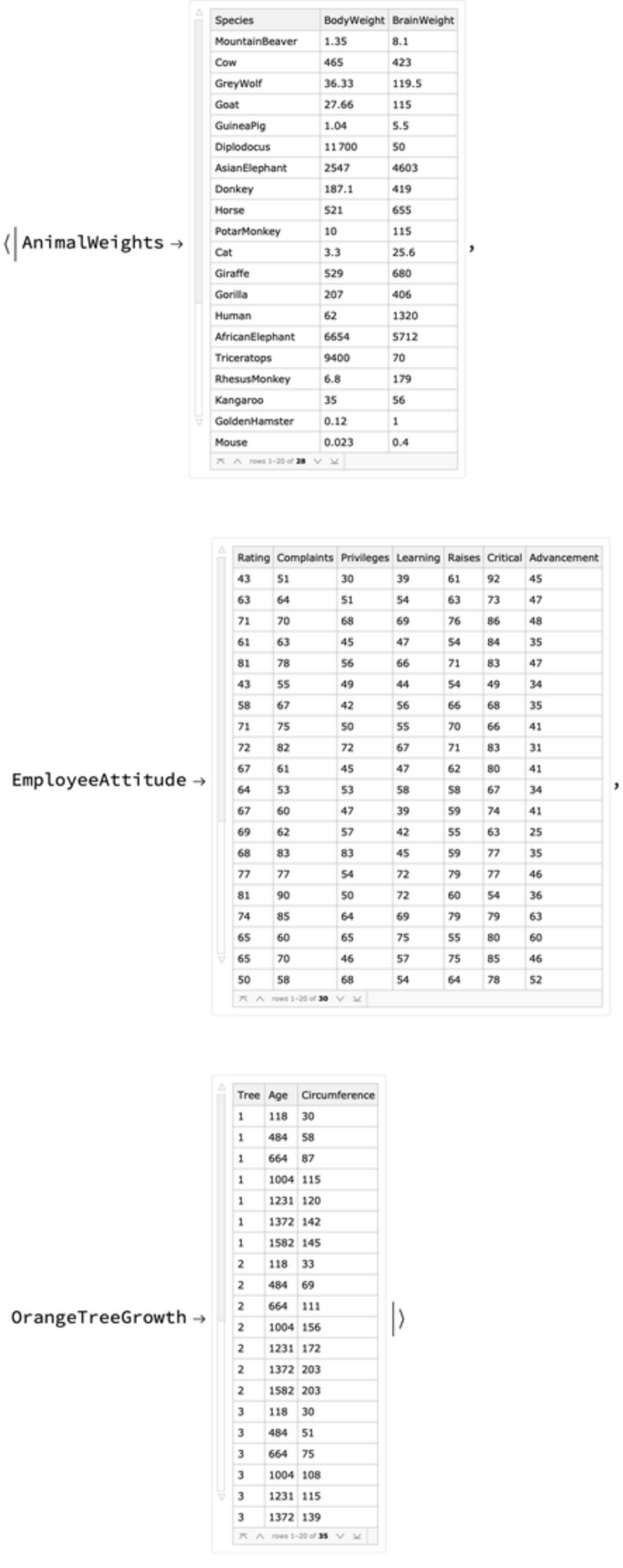Convert all datasets into long form datasets:

 In:=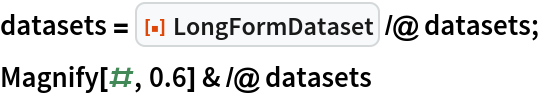Out=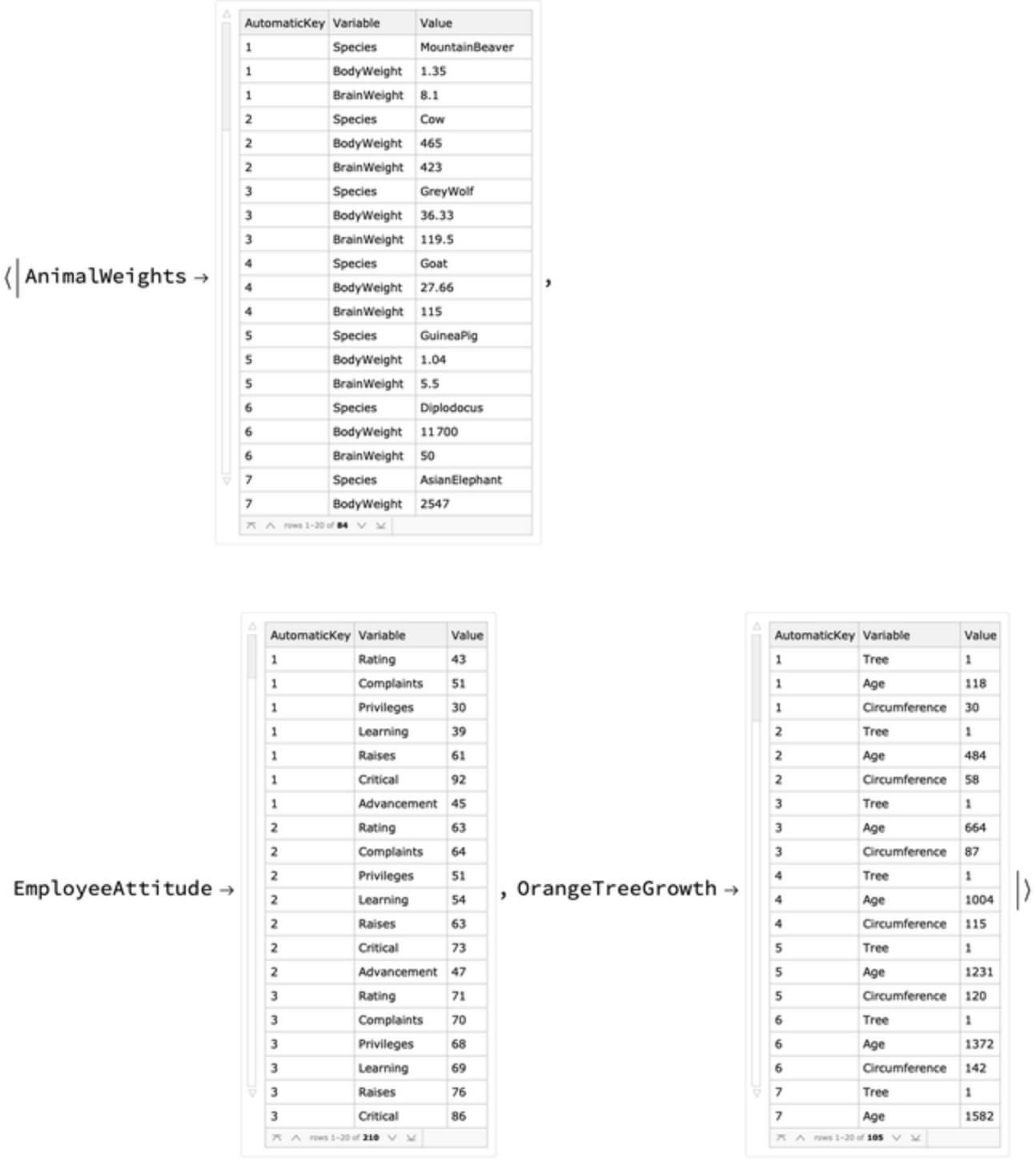For each long form dataset, change the automatic key column to include the name of that dataset:

 In:=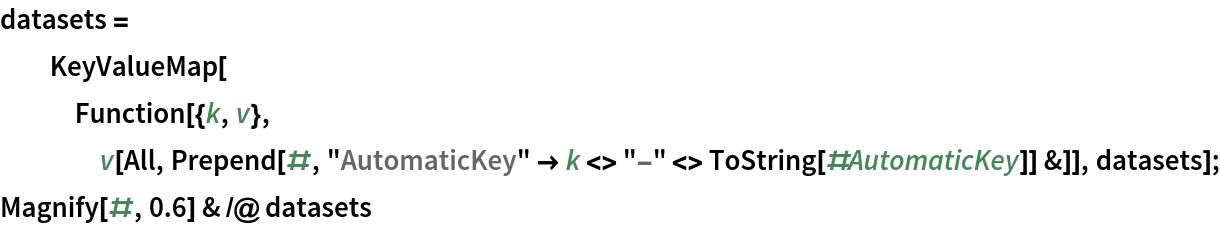Out=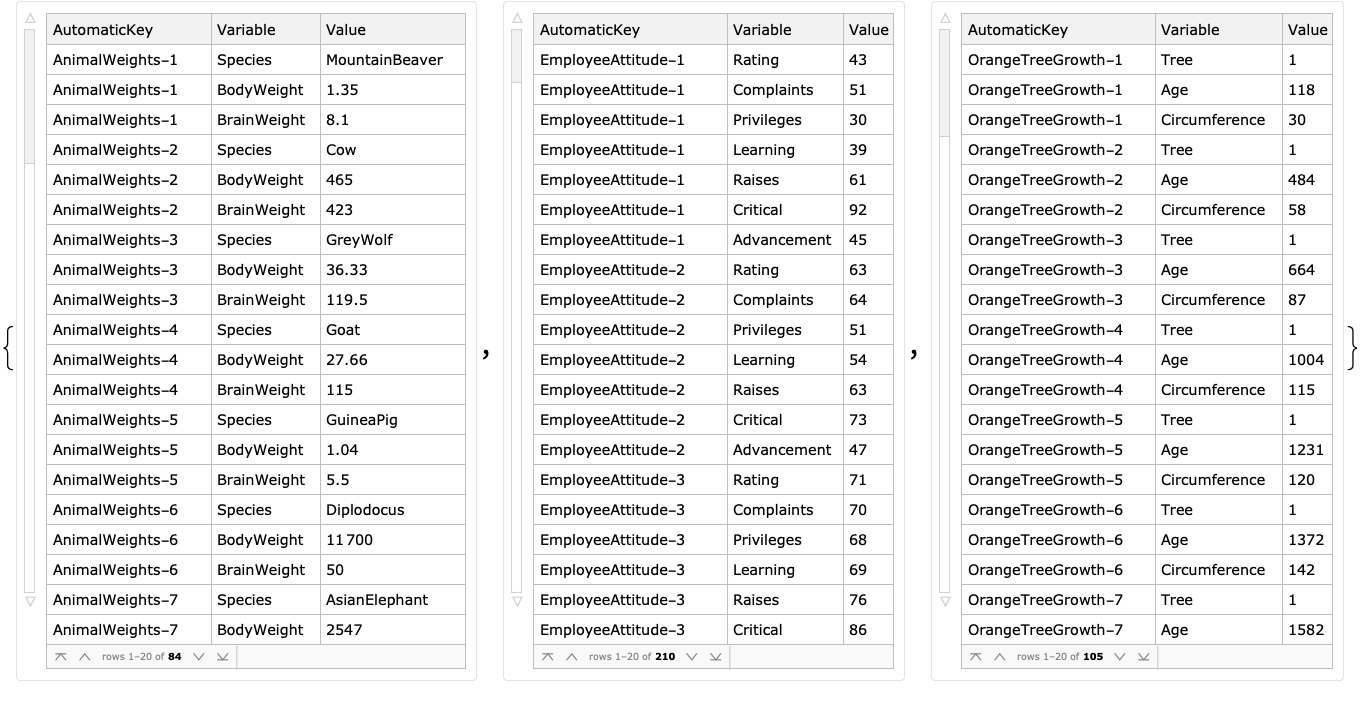Join all long form datasets into one dataset and show a sample:

 In:=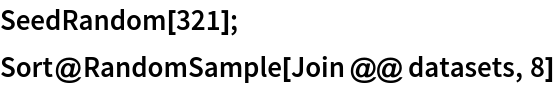Out=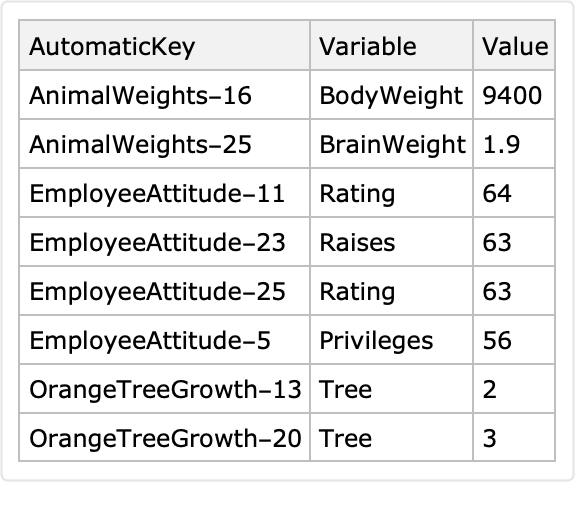### Properties and Relations (2)

Here is a random sparse matrix:

 In:=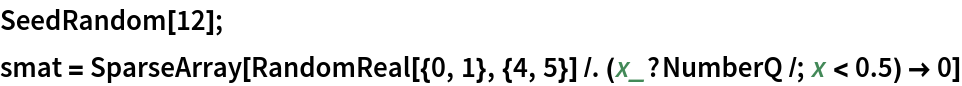Out=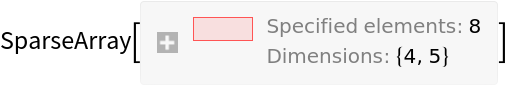Compare the array rules of this sparse matrix with its long-form representation:

 In:=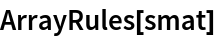Out=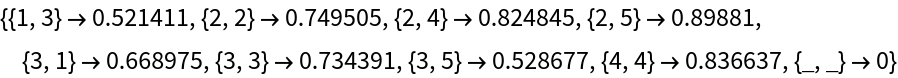Here is the long-form representation:

 In:=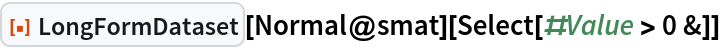Out=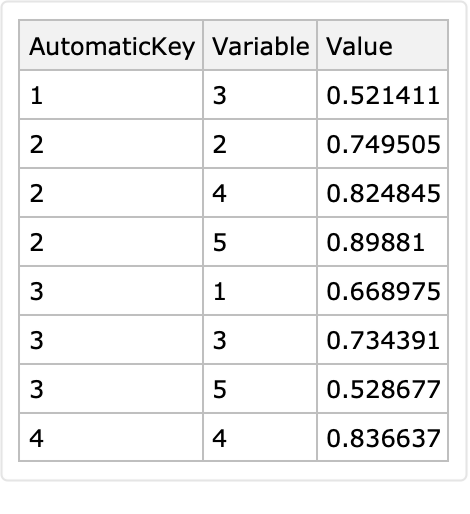Note that since using Normal on the sparse matrix makes a dense matrix with zeroes, those zeroes are filtered out from the displayed long form.

Cross tabulation can be seen under certain conditions as conversion to wide form. Therefore, certain long form conversions can be reverted into the original forms using cross tabulation.

Here is an example using the resource function over a subset of the Lake Mead elevation levels data:

 In:=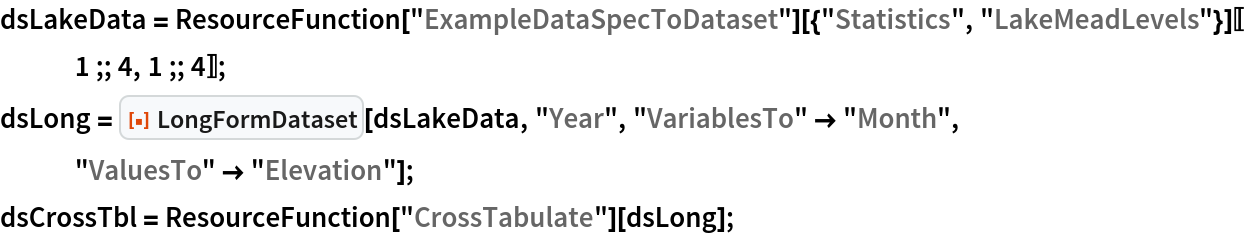In:=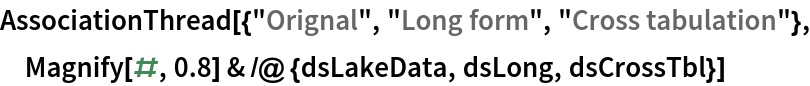Out=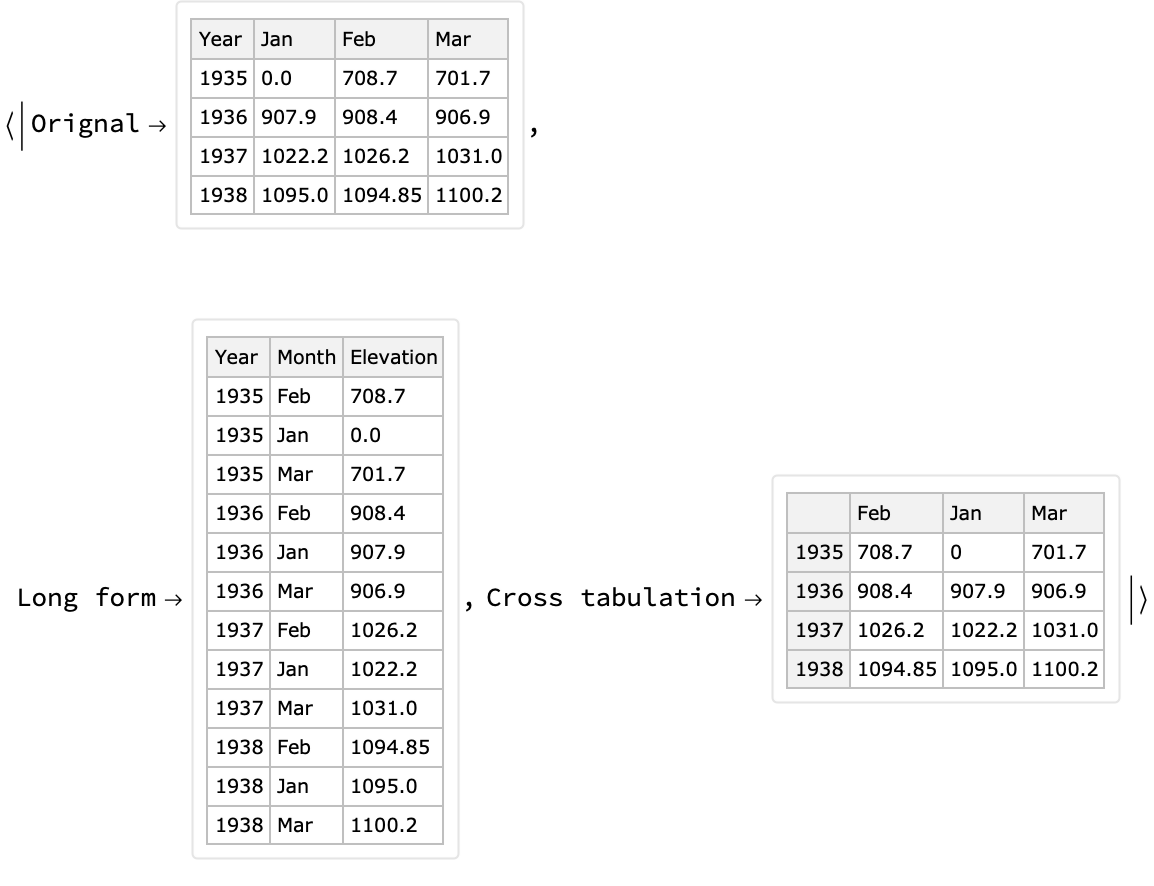### Possible Issues (2)

If the first argument is a dataset without column names, the second and third arguments are expected to consist of column indices.

Here is a correct specification:

 In:=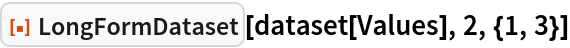Out=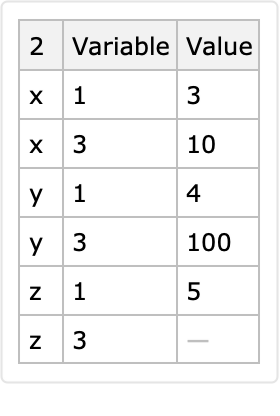Here are some incorrect specifications:

 In:=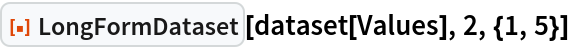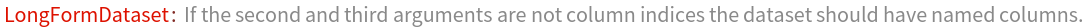Out=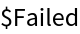In:=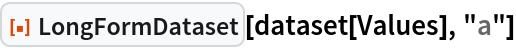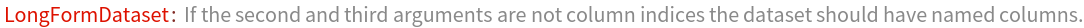Out=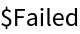If the identifier columns produce multiple corresponding rows for a given combination of identifier values, then only the last row is put in the long-form result:

 In:=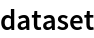Out=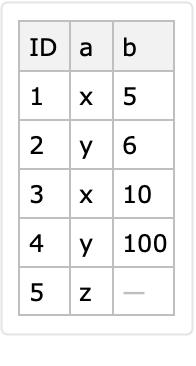The row <|"ID"1, "a" x, "b" 5|> is lost:

 In:=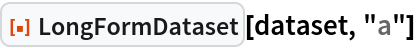Out=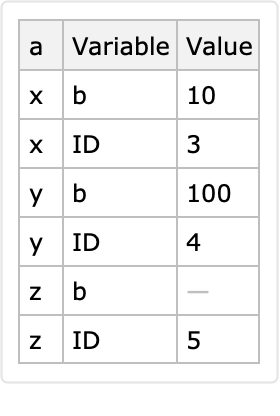## Author Notes

Conversions to long format and wide format are fundamental data transformation techniques.